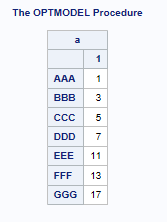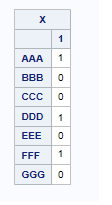## OptModel to find a variance in a table

I am trying to solve the classic find the number problem.

I have a report which has a variance on it of some value Z. I want to be able to pinpoint what rows may be the cause of the variance Z in my data. i.e there are certain rows that may add up to the Z. In a practical sense the situation plays out as follows:

Chad: "I received a listing of the balancing entries from Bill this afternoon, I'm not too sure what is making up the \$21 variance"

Bob: "Is it possible to find out what items are throwing out the variance? perhaps there are some entries that are unbalanced?"

Chad: (sigh) "I'll call my wife, I'm not going home tonight".

In most cases, Bob spends hours trying to find out which rows are causing the error, and enviably misses his dinner at home.

I have set up a sample problem with primes:

Items in "a" are primes indexed by the ID AAA,BBB,...etc.let's say I want to find out which numbers add up to 21. (answer is AAA + DDD + FFF)

I have constructed a vector X initialized as 0's and can only be binary values. My desired result is below:INPUT DATA = `OPTMODELSETUP `

```ID	Var1
AAA	1
BBB	3
CCC	5
DDD	7
EEE	11
FFF	13
GGG	17```

I'm not seeming to be able to get there:

``````proc optmodel;

/* declare index sets */
set <str> ListSet;
set VarChoices = 1..1;

/* declare parameters */
num a {ListSet,VarChoices} init 0;

/* read the set of Variables */
read data OPTMODELSETUP into ListSet=[ID] {j in VarChoices} <a[ID,j]=col('Var'||(j))>;

print a;

/* declare the model */
var X {ListSet, VarChoices} binary init 0;

min Objective = (sum {ID in ListSet, j in VarChoices} a[ID,j]*X[ID,j]);

con sum {j in VarChoices} a[ID,j]*X[ID,j] = 31;

/* call the solver and save the results */
solve;

/*ods output PrintTable#8=Solution;*/
print X;``````

Is this problem expandable such that all solutions can be displayed as different columns in X? we know in real life, there may be multiple solutions in which the rows total a certain value.

1 ACCEPTED SOLUTION

Accepted Solutions

## Re: OptModel to find a variance in a table

Here's one way to find a single solution (using 21 to match your example):

``````proc optmodel;
/* declare index sets */
set <str> ListSet;

/* declare parameters */
num a {ListSet} init 0;

/* read the set of Variables */
read data OPTMODELSETUP into ListSet=[ID] a=Var1;
print a;

/* declare the model */
var X {ListSet} binary;

con sum {ID in ListSet} a[ID]*X[ID] = 21;

/* call the solver and save the results */
solve noobj;
print X;
``````

To get all solutions and save them to a data set, you can do this:

``````   solve with clp / findallsolns;
create data out from [s]=(1.._NSOL_) {ID in ListSet} <col(ID)=(a[ID]*X[ID].sol[s])>;``````

That way outputs one observation per solution, but CREATE DATA can save the results however you want.  Here's a sparse alternative:

``   create data out2 from [s ID]={s in 1.._NSOL_, ID in ListSet: X[ID].sol[s] > 0.5} a=a[ID];``
3 REPLIES 3

## Re: OptModel to find a variance in a table

Here's one way to find a single solution (using 21 to match your example):

``````proc optmodel;
/* declare index sets */
set <str> ListSet;

/* declare parameters */
num a {ListSet} init 0;

/* read the set of Variables */
read data OPTMODELSETUP into ListSet=[ID] a=Var1;
print a;

/* declare the model */
var X {ListSet} binary;

con sum {ID in ListSet} a[ID]*X[ID] = 21;

/* call the solver and save the results */
solve noobj;
print X;
``````

To get all solutions and save them to a data set, you can do this:

``````   solve with clp / findallsolns;
create data out from [s]=(1.._NSOL_) {ID in ListSet} <col(ID)=(a[ID]*X[ID].sol[s])>;``````

That way outputs one observation per solution, but CREATE DATA can save the results however you want.  Here's a sparse alternative:

``   create data out2 from [s ID]={s in 1.._NSOL_, ID in ListSet: X[ID].sol[s] > 0.5} a=a[ID];``

## Re: OptModel to find a variance in a table

Nice one Rob!;
So if I'm interpreting the solution correctly,
s AAA BBB CCC DDD EEE FFF GGG
1 0 3 0 7 11 0 0
2 0 3 5 0 0 13 0
3 1 0 0 7 0 13 0
4 1 3 0 0 0 0 17

if we have any value in the column > 0 then we interpret that as a trigger?
i.e solution row 3 AAA + DDD + FFF
solution row 4 AAA + BBB + GGG = 21

Thanks Rob;

## Re: OptModel to find a variance in a table

Yes, that is the correct interpretation.

Discussion stats
• 3 replies
• 339 views
• 0 likes
• 2 in conversation